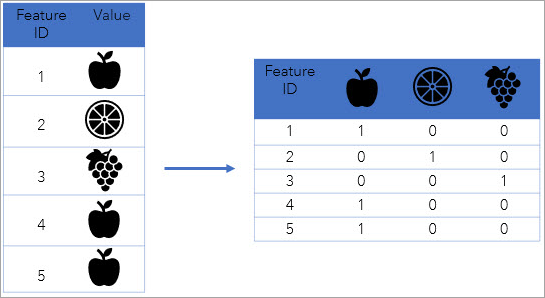# Encode Field (Data Management)

## Summary

Converts categorical values (string, integer, or date) into multiple numerical fields, each representing a category. The encoded numerical fields can be used in most data science and statistical workflows including regression models.

## Illustration## Usage

• The tool supports the following encoding methods:

• One-hot—Converts each categorical value into a new column and assigns 0 or 1. where 1 represents the presence of that categorical value.
• One-cold—Converts each categorical value into a new column and assigns 0 or 1. where 0 represents the presence of that categorical value.
• Temporal—Converts each date value in the field to encode into integer values (0, 1, 2, and so on) based on the time step interval. All the dates falling under the same time step interval are encoded together with the same integer. Three fields will be created if you use theTemporal method: a time step field that contains the encoded time steps, a start time field that contains the start time of the time interval, and an end time field that contains the end time of the time interval.

• The tool modifies the input data and appends the new encoded fields to the input table or feature class.

• When you choose the One-hot or One-cold method for the Encoding Method parameter, the number of fields will be equal to the number of categorical values in the field you choose to encode (including text and integer). If you use the Temporal encoding method, intervals of time steps are created based on the Time Step Interval parameter value and three fields are created containing the time step, start time, and end time.

• The Time Step Interval parameter is only applicable when the Temporal method is chosen for the Encoding Method parameter. The temporal value will be aggregated into a time step the time is within. The unit of the time step interval can be seconds, minutes, hours, days, weeks, months, or years.

• The Time Step Alignment parameter defines how aggregation will occur based on a given time step interval. The End time option aligns the time step to the last time event and aggregates back in time. The Start time option aligns time steps to the first-time event and aggregates forward in time. The Reference time option allows you to specify a particular date and time to which the time steps will be aligned.

## Syntax

`arcpy.management.EncodeField(in_table, field, {method}, {time_step_interval}, {time_step_alignment}, {reference_time})`
 Parameter Explanation Data Type in_table The input table or feature class containing the field to be encoded. Fields will be added to the existing input table and will not create a new output table. Table View; Raster Layer; Mosaic Layer field The field containing the categorical or temporal values to be encoded. Field method(Optional) Specifies the method to use to encode the values contained in the Field to Encode parameter.ONEHOT —Each categorical value will be converted to a new field and the values 0 and 1 will be assigned, where 1 represents the presence of that categorical value. This is the default.ONECOLD — Each categorical value will be converted to a new field and the values 0 and 1 will be assigned, where 0 represents the presence of that categorical value.TEMPORAL —Each temporal value in the Field to Encode parameter will be converted to an integer based on the time step interval, time step alignment, and reference time specified. String time_step_interval(Optional) The number of seconds, minutes, hours, days, weeks, or years that will represent a single time step. The temporal value will be aggregated into a certain time step it is within. If no value is provided, the default time step interval is based on two algorithms that are used to determine the optimal number and width of the time step intervals. The smaller of the two results is used as the time step interval. Time Unit time_step_alignment(Optional) Specifies how aggregation will occur based on the Time Step Interval parameter value.END_TIME — Time steps will align to the last time event and aggregate back in time. This is the default.START_TIME — Time steps will align to the first time event and aggregate forward in time.REFERENCE_TIME — Time steps will align to the date and time specified in the Reference Time parameter. Aggregation is performed forward and backward in time from the reference time until reaching the first and last temporal values. String reference_time(Optional) The date and time to which the time-step intervals will align. For example, to bin your data weekly from Monday to Sunday, set a reference time of Sunday at midnight to ensure that the time steps break between Sunday and Monday at midnight.The value can be a date and time or solely a date; it cannot be solely a time. The expected format is determined by the computer's regional time settings. Date

#### Derived Output

 Name Explanation Data Type updated_table The table that contains the added fields that were encoded. Table View

## Code sample

EncodeField example 1 (Python window)

The following Python window script demonstrates how to use the EncodeField tool.

``````arcpy.management.EncodeField("San_Francisco_Crimes",
"Category", "ONEHOT", '', None, "END_TIME")``````
EncodeField example 2 (stand-alone script)

The following stand-alone Python script demonstrates how to use the EncodeField tool.

``````# Import system modules.
import arcpy

try:
# Set the workspace and input features.
arcpy.env.workspace = r"C:\\Encoded\\MyData.gdb"
inputFeatures = 'San_Francisco_Crimes'

# Set input features, dependent variable, and explanatory variable.
in_table = 'San_Francisco_Crimes'
field = 'Dates'

# Set encoding Method
encoding_method = "TEMPORAL"

# Set time Step Interval
time_step_interval = '1 Days'

# Set Time Step Alignment
time_step_alignment = "START_TIME"

# Run Encode Field Tool.
arcpy.management.EncodeField(in_table, field, encoding_method,
None, time_step_interval, time_step_alignment)

except arcpy.ExecuteError:
# If an error occurred when running the tool, print the error message.
print(arcpy.GetMessages())``````

## Licensing information

• Basic: Yes
• Standard: Yes